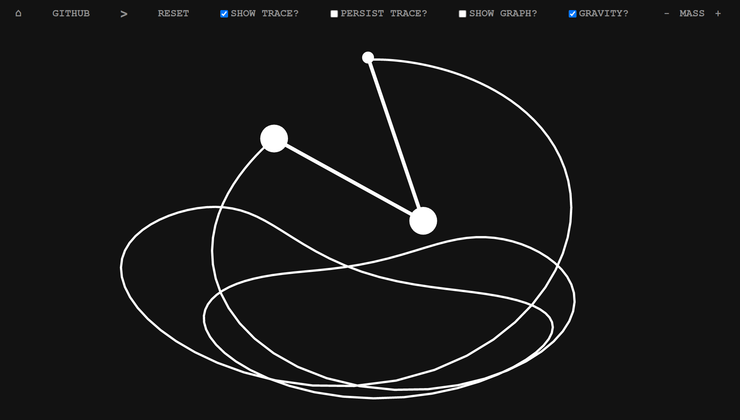## CodeNewbie Community is a community of 10,715 amazing newbies# Interactive Double Pendulum Playground Using SVG

A double pendulum is a pendulum with another pendulum attached to its end, is a simple physical system that exhibits rich dynamic behavior with a strong sensitivity to initial conditions.

The motion of a double pendulum is governed by a set of coupled ordinary differential equations and is chaotic.

 θ1'' = −g (2 m1 + m2) sin θ1 − m2 g sin(θ1 − 2 θ2) − 2 sin(θ1 − θ2) m2 (θ2'2 L2 + θ1'2 L1 cos(θ1 − θ2)) L1 (2 m1 + m2 − m2 cos(2 θ1 − 2 θ2))
 θ2'' = 2 sin(θ1 − θ2) (θ1'2 L1 (m1 + m2) + g(m1 + m2) cos θ1 + θ2'2 L2 m2 cos(θ1 − θ2)) L2 (2 m1 + m2 − m2 cos(2 θ1 − 2 θ2))